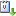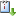Usted está aquí: "Risk minimization and optimal derivative design in a principal-agent game" - Santiago Moreno Bromberg (University of British Columbia)

# "Risk minimization and optimal derivative design in a principal-agent game" - Santiago Moreno Bromberg (University of British Columbia)

Ponente:
Cuándo 10/04/2008 de 11:00 a 12:00 Salón "Graciela Salicrup"vCaliCal

### Resumen:

We study a Principal-Agent model of optimal derivative design where the agents' preferences are of mean-variance type and their types characterize their risk aversion. The set of contracts traded expose the principal to additional risk as measures by a convex risk measure in exchange for a known revenue.

The principal's aim is to minimize her risk exposure by trading with the agents subject to the standard incentive compatibility and individual rationality conditions on the agents' choices. In order to prove that the principal's risk minimization problem has a solution, we first follow the seminal idea of Rochet and Choné and characterize incentive compatible catalogues in terms of U-convex functions. When the impact of a single trade on the principal's revenues is linear as in the recent paper by Carlier, Ekeland and Touzi, the link between incentive compatibility and U-convexity is key to establish the existence of an optimal solution. In our model the impact is non-linear as a single trade has a non-linear impact on the principal's risk assessment. Due to this non-linearity we face a non-standard variational problem where the objective cannot be written as the integral of a given Lagrangian. Instead, our problem can be decomposed into a standard variational part representing the aggregate income of the principal, plus the minimization of the principal's risk evaluation, which depends on the aggregate of the derivatives traded.